# Improved WD Lemma Generation

## Contents

This page describes work done for optimising well-definedness lemmas generated by the Core Rodin platform.

The lemmas are generated according to the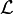$\mathcal{L}$ operator defined in Well-definedness in Event-B. However, this operator being defined as a syntactic transformation, it sometimes generates lemmas that are unnecessarily complicated. We first give some motivating examples showing cases where this happens, then we describe what result shall be produced by the tool. Finally, we describe the design retained for development.

## Motivating Examples

All examples corresponds to the behavior of Rodin 1.3

### Example A

The well-definedness lemma generated for predicate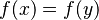$f(x) = f(y)$ is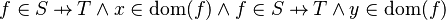$f\in S\pfun T\land x\in\dom(f)\land f\in S\pfun T\land y\in\dom(f)$


This predicate is sub-optimal as it contains twice the same sub-predicate (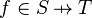$f\in S\pfun T$). Consequently, when the prover is fed with the generated lemma, it will have to prove twice the same goal.

### Example B

The well-definedness lemma generated for predicate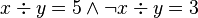$x \div y=5 \land \lnot x \div y=3$ is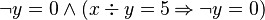$\lnot y=0 \land (x\div y=5 \limp \lnot y=0)$


This predicate is sub-optimal as the sub-predicate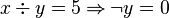$x\div y=5 \limp \lnot y=0$ is subsumed by the sub-predicate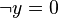$\lnot y=0$. The prover doesn't need to prove$x\div y=5 \limp \lnot y=0$ if$\lnot y=0$ has been proved.

### Example C

In the well-definedness lemma,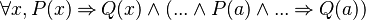$\forall x, P(x) \limp Q(x) \land (... \land P(a) \land ... \limp Q(a))$,

the sub-predicate$(... \land P(a) \land ... \limp Q(a))$ is subsumed by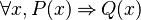$\forall x, P(x) \limp Q(x)$.

In the same way, in the well-definedness lemma,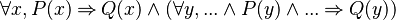$\forall x, P(x) \limp Q(x) \land (\forall y, ... \land P(y) \land ... \limp Q(y))$,

the sub-predicate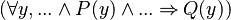$(\forall y,... \land P(y) \land ... \limp Q(y))$ is subsumed by$\forall x, P(x) \limp Q(x)$.

This issue will not be fixed by the improved generation.

### Example D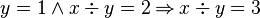$y = 1 \land x \div y = 2 \limp x \div y = 3$ will produce the well-definedness lemma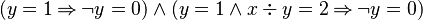$(y = 1 \limp \lnot y = 0) \land (y = 1 \land x \div y = 2 \limp \lnot y = 0 )$. In this well-definedness lemma, the sub-predicate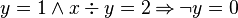$y = 1 \land x \div y = 2 \limp \lnot y = 0$ is subsumed by the sub-predicate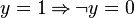$y = 1 \limp \lnot y = 0$. In the first implication, the consequent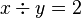$x \div y = 2$ is unnecessary.

## Improved Generation

To fix the issues shown in the above examples, we need that the tool produces a WD lemma equivalent (in the logical sense) to the predicate obtained through the$\mathcal{L}$ operator and satisfying the following constraint : No sub-predicate subsumes another sub-predicate (exception : example C).

The constraint covers examples A, B and D.

## Design for Improved Generation

The old mechanism for Well-Definedness lemma generation has been externalized using Design Pattern Visitor. This mechanism is still used to generate an unsimplified Well-Definedness lemma. To simplify this Well-Definedness lemma, we use the Design Pattern Visitor to create a new tree made up of predicates, implications, conjunctions and universal quantifiers, while traversing the whole AST. This tree will be simplified by removing redundant predicates and using optimizations, and will be used to build a simplified Well-Definedness lemma. This visitor will be applicable to all predicates. As some Well-Definedness lemmas will be simplified, some WD proofs will be broken.

All the classes created for Well-Definedness generation can be found in the package "org.eventb.internal.core.ast.wd".